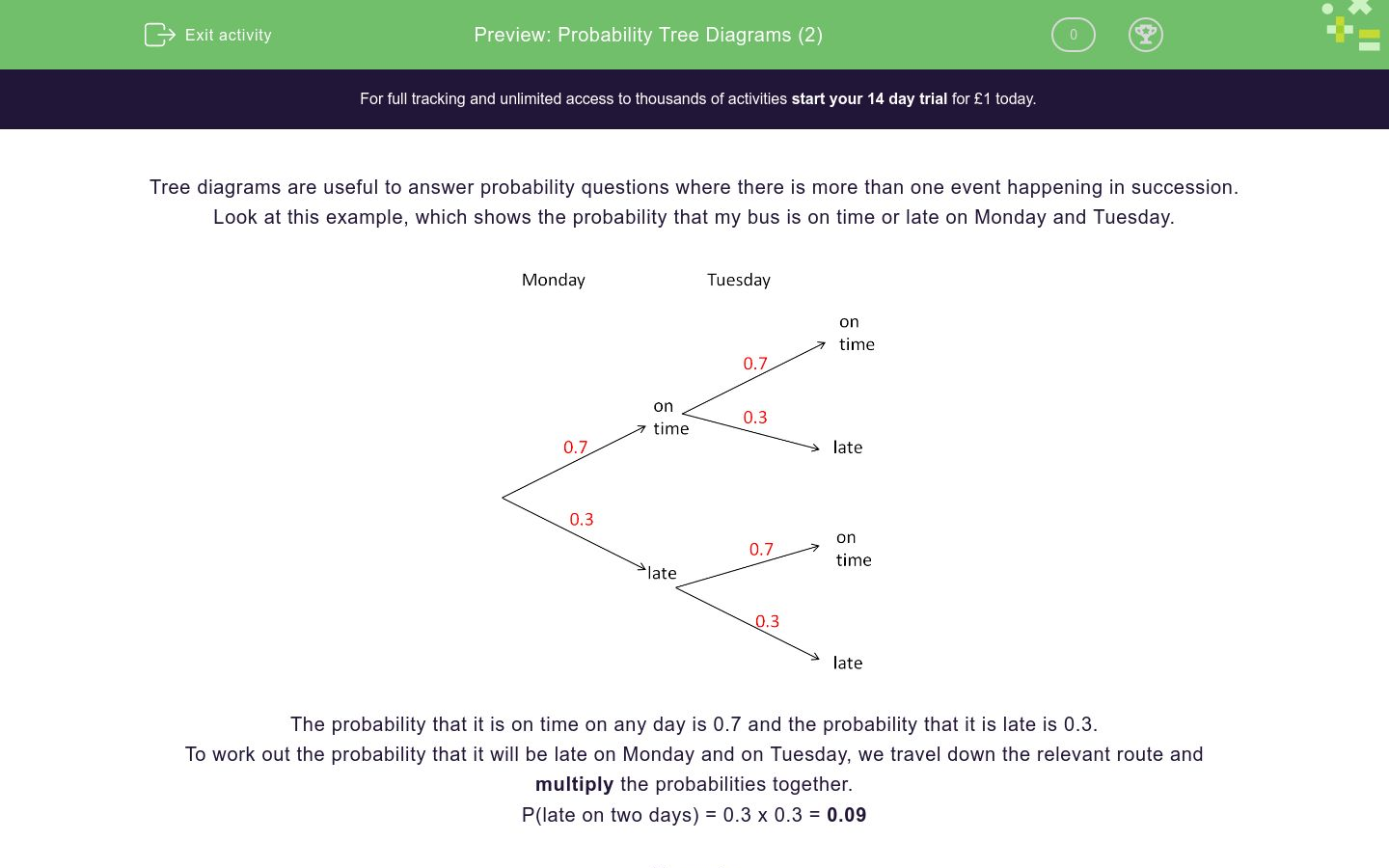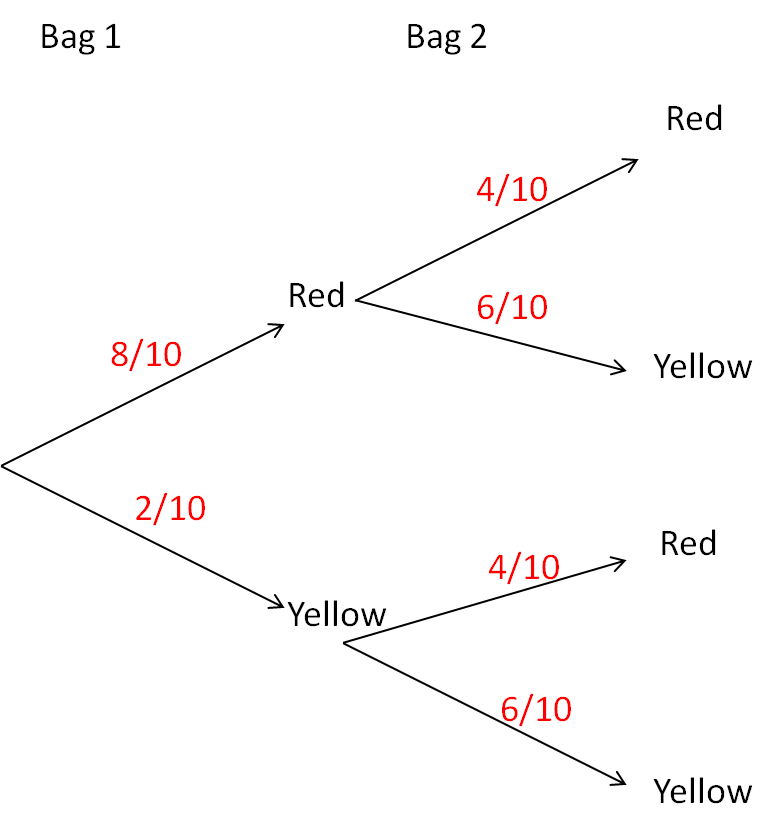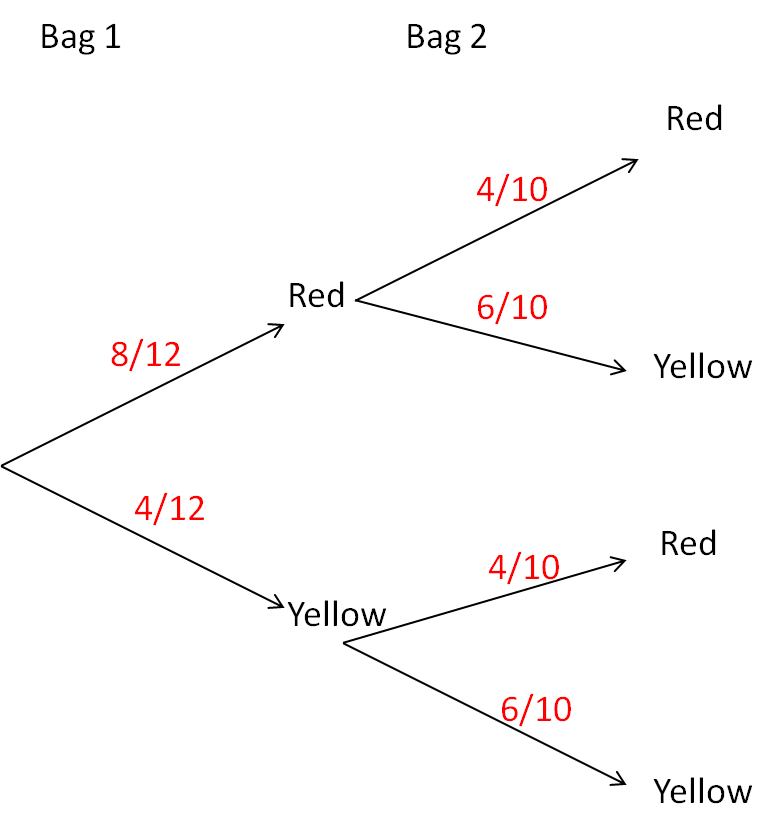# Probability Tree Diagrams (2)

In this worksheet, students understand and use two-stage tree diagrams to answer probability questions.Key stage:  KS 3

Curriculum topic:   Probability

Curriculum subtopic:   Calculate Theoretical Probability

Difficulty level:### QUESTION 1 of 10

Tree diagrams are useful to answer probability questions where there is more than one event happening in succession.

Look at this example, which shows the probability that my bus is on time or late on Monday and Tuesday.The probability that it is on time on any day is 0.7 and the probability that it is late is 0.3.

To work out the probability that it will be late on Monday and on Tuesday, we travel down the relevant route and multiply the probabilities together.

P(late on two days) = 0.3 x 0.3 = 0.09

Example

There are 8 red balls and 2 yellow balls in Bag 1.

There are 4 red balls and 6 yellow balls in Bag 2.

I take one ball from Bag 1 and then one ball from Bag 2.

What is the probability of choosing two red balls.

Here is a tree diagram to show this information.P(Red Red) = 8/10 x 4/10 = 32/100 = 8/25

There are 8 red balls and 2 yellow balls in Bag 1.

There are 4 red balls and 6 yellow balls in Bag 2.

I take one ball from Bag 1 and then one ball from Bag 2.

What is the probability of choosing two yellow balls.There are 8 red balls and 2 yellow balls in Bag 1.

There are 4 red balls and 6 yellow balls in Bag 2.

I take one ball from Bag 1 and then one ball from Bag 2.

What is the probability of choosing a red ball first and then a yellow ball.There are 8 red balls and 2 yellow balls in Bag 1.

There are 4 red balls and 6 yellow balls in Bag 2.

I take one ball from Bag 1 and then one ball from Bag 2.

What is the probability of choosing a red ball and a yellow ball in any order.There are 8 red balls and 4 yellow balls in Bag 1.

There are 4 red balls and 6 yellow balls in Bag 2.

I take one ball from Bag 1 and then one ball from Bag 2.

What is the probability of choosing two yellow balls.There are 8 red balls and 4 yellow balls in Bag 1.

There are 4 red balls and 6 yellow balls in Bag 2.

I take one ball from Bag 1 and then one ball from Bag 2.

What is the probability of choosing two red balls.There are 8 red balls and 4 yellow balls in Bag 1.

There are 4 red balls and 6 yellow balls in Bag 2.

I take one ball from Bag 1 and then one ball from Bag 2.

What is the probability of choosing one red ball and one yellow ball in any order.There are 7 red balls and 5 yellow balls in Bag 1.

There are 6 red balls and 4 yellow balls in Bag 2.

I take one ball from Bag 1 and then one ball from Bag 2.

What is the value of the probability marked A?There are 7 red balls and 5 yellow balls in Bag 1.

There are 6 red balls and 4 yellow balls in Bag 2.

I take one ball from Bag 1 and then one ball from Bag 2.

What is the value of the probability marked B?There are 7 red balls and 5 yellow balls in Bag 1.

There are 6 red balls and 4 yellow balls in Bag 2.

I take one ball from Bag 1 and then one ball from Bag 2.

What is the probability that I select two red balls?There are 7 red balls and 5 yellow balls in Bag 1.

There are 6 red balls and 4 yellow balls in Bag 2.

I take one ball from Bag 1 and then one ball from Bag 2.

What is the probability that I select two yellow balls?• Question 1

There are 8 red balls and 2 yellow balls in Bag 1.

There are 4 red balls and 6 yellow balls in Bag 2.

I take one ball from Bag 1 and then one ball from Bag 2.

What is the probability of choosing two yellow balls.3/25
• Question 2

There are 8 red balls and 2 yellow balls in Bag 1.

There are 4 red balls and 6 yellow balls in Bag 2.

I take one ball from Bag 1 and then one ball from Bag 2.

What is the probability of choosing a red ball first and then a yellow ball.12/25
• Question 3

There are 8 red balls and 2 yellow balls in Bag 1.

There are 4 red balls and 6 yellow balls in Bag 2.

I take one ball from Bag 1 and then one ball from Bag 2.

What is the probability of choosing a red ball and a yellow ball in any order.14/25
EDDIE SAYS
56/100
• Question 4

There are 8 red balls and 4 yellow balls in Bag 1.

There are 4 red balls and 6 yellow balls in Bag 2.

I take one ball from Bag 1 and then one ball from Bag 2.

What is the probability of choosing two yellow balls.1/5
EDDIE SAYS
24/120
• Question 5

There are 8 red balls and 4 yellow balls in Bag 1.

There are 4 red balls and 6 yellow balls in Bag 2.

I take one ball from Bag 1 and then one ball from Bag 2.

What is the probability of choosing two red balls.4/15
EDDIE SAYS
32/120
• Question 6

There are 8 red balls and 4 yellow balls in Bag 1.

There are 4 red balls and 6 yellow balls in Bag 2.

I take one ball from Bag 1 and then one ball from Bag 2.

What is the probability of choosing one red ball and one yellow ball in any order.8/15
EDDIE SAYS
48/120 + 16/120 = 64/120
• Question 7

There are 7 red balls and 5 yellow balls in Bag 1.

There are 6 red balls and 4 yellow balls in Bag 2.

I take one ball from Bag 1 and then one ball from Bag 2.

What is the value of the probability marked A?7/12
• Question 8

There are 7 red balls and 5 yellow balls in Bag 1.

There are 6 red balls and 4 yellow balls in Bag 2.

I take one ball from Bag 1 and then one ball from Bag 2.

What is the value of the probability marked B?4/10
• Question 9

There are 7 red balls and 5 yellow balls in Bag 1.

There are 6 red balls and 4 yellow balls in Bag 2.

I take one ball from Bag 1 and then one ball from Bag 2.

What is the probability that I select two red balls?7/20
EDDIE SAYS
42/120
• Question 10

There are 7 red balls and 5 yellow balls in Bag 1.

There are 6 red balls and 4 yellow balls in Bag 2.

I take one ball from Bag 1 and then one ball from Bag 2.

What is the probability that I select two yellow balls?1/6
EDDIE SAYS
20/120
---- OR ----

Sign up for a £1 trial so you can track and measure your child's progress on this activity.

### What is EdPlace?

We're your National Curriculum aligned online education content provider helping each child succeed in English, maths and science from year 1 to GCSE. With an EdPlace account you’ll be able to track and measure progress, helping each child achieve their best. We build confidence and attainment by personalising each child’s learning at a level that suits them.

Get started